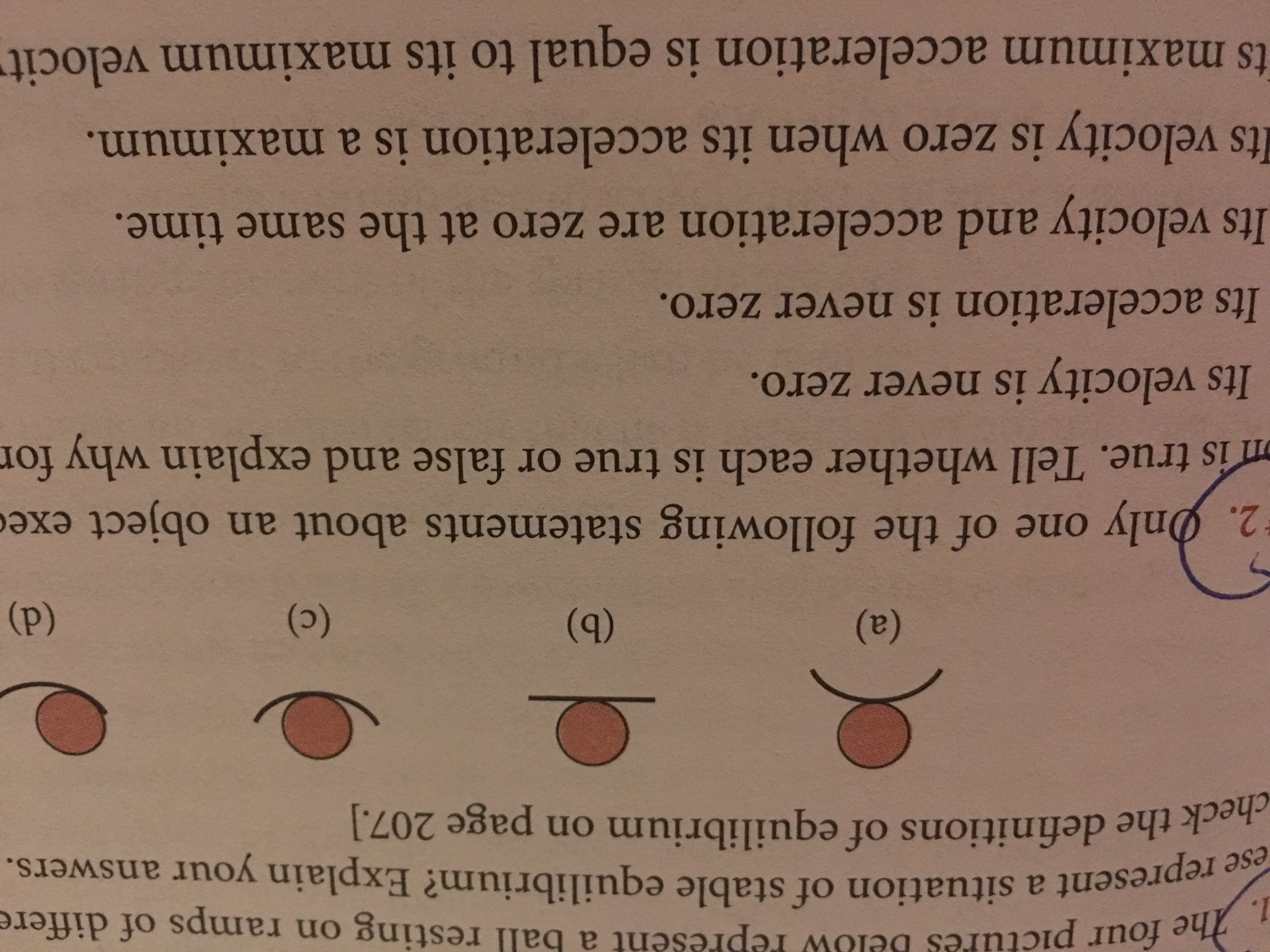# ures below Pepresent a ball resting on ramps of differetherepresent a situation of stable equilibrium? Explain your answers.eesecheck the definitions of equilibrium on page 207.]2·hnly one of the following statements about an object exeis true. Tell whether each is true or false and explain why forIts velocity is never zero.Its acceleration is never zero.Its velocity and acceleration are zero at the same timeIts velocity is zero when its acceleration is a maximumts maximum acceleration is equal to its maximum velocit

Question
57 views

Only one of the following statements about an object executing simple harmonic motion is true. Tell whether each is true or false and explain why for each.help_outlineImage Transcriptioncloseures below Pepresent a ball resting on ramps of differe the represent a situation of stable equilibrium? Explain your answers. e ese check the definitions of equilibrium on page 207.] 2·hnly one of the following statements about an object exe is true. Tell whether each is true or false and explain why for Its velocity is never zero. Its acceleration is never zero. Its velocity and acceleration are zero at the same time Its velocity is zero when its acceleration is a maximum ts maximum acceleration is equal to its maximum velocit fullscreen
check_circle

Step 1

Simple harmonic motion is described as,

Step 2

Acceleration is a function of sine. Whereas, velocity is a function of cosine. When sine is maximum, cosine is minimum. Therefore, only 4th statement is true.

Step 3

Velocity can be zero when cosine term is zero. Acceleration is zero at t = 0. Acceleration and velocity can never be zero at the same time since they are different ...

### Want to see the full answer?

See Solution

#### Want to see this answer and more?

Solutions are written by subject experts who are available 24/7. Questions are typically answered within 1 hour.*

See Solution
*Response times may vary by subject and question.
Tagged in

### Kinematics